Published: 09 October 2015

# Finite element analysis of coupled vibration for hoisting cable with time-varying length

Jinjie Wang1
Guohua Cao2
Mingxing Lin3
Shanzeng Liu4
1, 2Jiangsu Key Laboratory of Mine Mechanical and Electrical, Xuzhou, China
1, 2, 4School of Mechatronic Engineering, China University of Mining and Technology, Xuzhou, China
3School of Mechanical Engineering, Shandong University, Jinan, China
Corresponding Author:
Guohua Cao
Views 23

#### Abstract

The coupled axial-torsional responses of the hoisting cable with time-varying length are investigated in order to predict the longitudinal vibration more accurately. The equations of motion are formulated by Hamilton’s principle and the finite element method (FEM), in which a variable-length cable element is introduced. In order to validate this theoretical model, an ADAMS simulation model is established in the framework of the multi-body system dynamic. The result shows that the numerical solution is in reasonably good agreement with the ADAMS simulation. The frequencies of the cables with the coupling considered and neglected are analyzed by varying the excitation frequency, which indicates that the coupling effect reduces the natural frequency of the cable and the maximum amplitude shifts from the resonance region to the deceleration stage as the coupling coefficient increases.

## 1. Introduction

Cables, due to their light weight and their ability to resist relatively large axial loads, have been extensively employed in diverse engineering applications [1-3]. However, they are subjected to large-amplitude vibrations for their high flexibility and low internal damping. Thus, the dynamic behavior of cables has been studied widely for decades. Recently, the coupled vibration of the cable with time-varying length has attracted a great deal of attention and various approaches have been proposed. Wang et al.  analyzed the coupled lateral- transverse-longitudinal dynamics of an underwater, drawn cable with an attached mass. A variable-domain element is adopted to discretize the equations of motion. Later, Kaczmarczyk and Ostachowicz  exhibited the coupled lateral-longitudinal dynamic response of the catenary-vertical ropes in the deep mine hoisting system and obtained the numerical solution by using the Rayleigh-Ritz method. Zhang et al.  presented the coupled lateral-longitudinal vibration model of the vertically translating elevator cables subjected to the general initial conditions and the external excitation with Galerkin method.

Cables are characterized by the coupled axial-torsional behavior when subjected to the external loads. Therefore, the vibration analysis of the coupled axial-torsional cable is of great significance. Samras  tested experimentally the coupling coefficients and discovered that the axial-torsional stiffness coefficient is approximately equal to the torsional-axial stiffness coefficient. Hashemi and Roach  presented the derivation of a dynamic finite element for the coupled extension-torsion vibration analysis of the cable and evaluated the coupled frequencies.

However, few researchers concentrated on the coupled axial-torsional dynamic responses, caused by the external displacement excitation, of the hoisting cable with time-varying length. Some problems have arisen in the hoisting cable of the mine rescue capsule shown in Fig. 1(a). On one hand, the torsion of hoisting cable is easy to destroy the electric cables wound around the surface of the hoisting cable; on the other hand, the torsion of cable aggravates the longitudinal vibration of itself and brings about the slight rotation of the capsule. These two facts reduce the performance of rescue capsules greatly both on safety and comfort. Therefore, it is significant to recognize the essential difference between the cables with the coupling considered and neglected.

This paper is organized as follows. In Section 2, the finite element model is established by adopting a variable-length element. For purpose of verifying the theoretical model, Section 3 presents the virtual prototype of the hoisting system established by using the ADAMS software package. In Section 4, t the results of the axial and torsional responses from both simulations are compared and the frequencies of the cables with the coupling included and neglected are analyzed. Finally, Section 5 draws some conclusions.

Fig. 1Hoisting system with time-varying length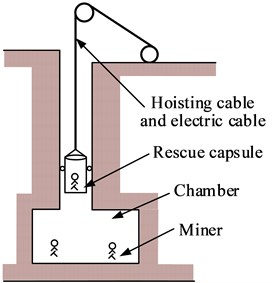a) Mine rescue capsule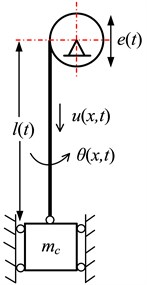b) Schematic diagram

## 2. Theoretical investigation

For simplicity, the hoisting system can be modelled as one vertically translating model described by a cylindrical coordinate system. As illustrated in Fig. 1(b), the hoisting cable of length $l\left(t\right)$ at time instant $t$ is wound on a head sheave at the top end and is attached with a mass ${m}_{c}$ at the lower end. The harmonic excitation $e\left(t\right)=A\mathrm{s}\mathrm{i}\mathrm{n}\left(\omega t\right)$ at position $x=$0 caused by the out-of-round head sheave should be considered because of resonance.

With reference to Fig. 1(b), the kinetic energy $T$ of hoisting cable can be expressed as:

1
$T=\frac{1}{2}{\int }_{0}^{l\left(t\right)}\left[\rho {\left(\frac{Du}{Dt}+v\right)}^{2}+J{\left(\frac{D\theta }{Dt}\right)}^{2}\right]dx,$

where $\rho$ and $J$ are the mass per unit length and moment of inertia of the hoisting cable.

The total potential energy $V$ of the system can be represented as:

2
$V={\int }_{0}^{l\left(t\right)}\frac{1}{2}\left[\left({Q}_{1}\epsilon +{Q}_{2}\varphi \right)\epsilon +\left({Q}_{3}\epsilon +{Q}_{4}\varphi \right)\varphi \right]dx,$

where ${Q}_{1}$, ${Q}_{2}$, ${Q}_{3}$ and ${Q}_{4}$ are the axial, axial-torsional coupling, torsional-axial coupling, and torsional stiffness coefficients of the hoisting cable, respectively. The approximation ${Q}_{23}={Q}_{2}={Q}_{3}$ will be adopted in the following derivation . The strain $\epsilon$ and $\varphi$ can be defined as $\epsilon ={u}_{x}$, $\varphi ={\theta }_{x}$. The virtual work is done by the interaction force $H$ can be expressed as:

3
$\delta W=-H\cdot \delta u\left(l,t\right)=-{m}_{c}\left(\frac{{D}^{2}u}{D{t}^{2}}+a\right)\cdot \delta u\left(l,t\right).$

As shown in Fig. 2, the whole hoisting cable is divided into a number of cable elements. Every cable element presented here consists of two nodes and each node has the axial displacement $u\left(x,t\right)$ and torsional deformation $\theta \left(x,t\right)$. It should be noted that the variable-length element  is adopted since the length of the hoisting cable varies with time. However, as opposed to the length, the number of elements $n$ remains constant.

Fig. 2Cable element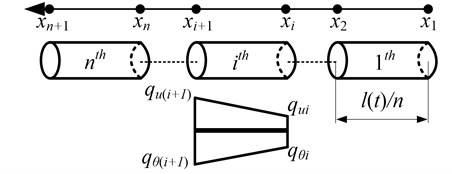In the FEM, the cable displacements could be approximated by the discretized nodal displacements. Thus, the displacements of any point within the $i$th element can be related to the discretized nodal displacement vectors ${\mathbf{q}}_{ui}$ and ${\mathbf{q}}_{\theta i}$ as well as the shape function matrices ${\mathbf{N}}_{i}$ as:

4
$u\left(x,t\right)={\mathbf{N}}_{i}\left(x,l\left(t\right)\right){\mathbf{q}}_{ui}\left(t\right),\theta \left(x,t\right)={\mathbf{N}}_{i}\left(x,l\left(t\right)\right){\mathbf{q}}_{\theta i}\left(t\right),$

where ${\mathbf{q}}_{ui}$ and ${\mathbf{q}}_{\theta i}$ are the 2×1 nodal displacement vectors and given by $\left[\begin{array}{ll}{q}_{ui}\left(t\right)& {q}_{u\left(i+1\right)}\left(t\right)\end{array}{\right]}^{T}$ and $\left[\begin{array}{ll}{q}_{\theta i}\left(t\right)& {q}_{\theta \left(i+1\right)}\left(t\right)\end{array}{\right]}^{T}$. ${\mathbf{N}}_{i}$ is derived from the linear displacement of element and defined as:

5
${\mathbf{N}}_{i}=\left[\frac{{x}_{i+1}-x}{{x}_{i+1}-{x}_{i}},\frac{x-{x}_{i}}{{x}_{i+1}-{x}_{i}}\right]=\frac{n}{l}\left[\frac{l}{n}i-x,x-\frac{l}{n}\left(i-1\right)\right].$

Differentiating Eq. (5) with respect to $x$ and $t$ yields:

6
${\mathbf{N}}_{ix}=\frac{n}{l}\left[-1,1\right],\mathrm{}\mathrm{}\mathrm{}{\mathbf{N}}_{it}=\frac{nv}{{l}^{2}}\left[x,-x\right],\mathrm{}\mathrm{}\mathrm{}{\mathbf{N}}_{ixt}=\frac{nv}{{l}^{2}}\left[-1,1\right],\mathrm{}\mathrm{}\mathrm{}{\mathbf{N}}_{itt}=\left(la-2{v}^{2}\right)\frac{n}{{l}^{3}}\left[x,-x\right].$

Also, the variations of $u\left(x,t\right)$ and $\theta \left(x,t\right)$ are expressed as:

7
$\delta u=\delta {\mathbf{q}}_{ui}^{T}{\mathbf{N}}_{i}^{T},\mathrm{}\mathrm{}\mathrm{}\delta \theta =\delta {\mathbf{q}}_{\theta i}^{T}{\mathbf{N}}_{i}^{T},\mathrm{}\mathrm{}\mathrm{}\delta {u}_{x}=\delta {\mathbf{q}}_{ui}^{T}{\mathbf{N}}_{ix}^{T},\mathrm{}\mathrm{}\mathrm{}\delta {\theta }_{x}=\delta {\mathbf{q}}_{\theta i}^{T}{\mathbf{N}}_{ix}^{T}.$

Substituting Eqs. (1)-(3) into the Hamilton’s principle and applying the variation operation can obtain that:

8
${\int }_{{t}_{1}}^{{t}_{2}}\sum _{i=1}^{n}\delta {T}_{i}dt={\int }_{t1}^{{t}_{2}}\sum _{i=1}^{n}{\int }_{{x}_{i}}^{{x}_{i+1}}\left\{\rho \left[\left({v}^{2}+v{u}_{t}+{v}^{2}{u}_{x}\right)\delta {u}_{x}-\left(a+{u}_{tt}+a{u}_{x}+v{u}_{xt}\right)\right\\delta u\right]$
$+J\left[\left(v{u}_{t}+{v}^{2}{u}_{x}\right)\delta {\theta }_{x}-\left({u}_{tt}+a{u}_{x}+v{u}_{xt}\right)\delta \theta \right]}dxdt,$
9
${\int }_{{t}_{1}}^{{t}_{2}}\sum _{i=1}^{n}\delta {V}_{i}dt={\int }_{t1}^{{t}_{2}}\sum _{i=1}^{n}{\int }_{{x}_{i}}^{{x}_{i+1}}\left[\left({Q}_{1}{u}_{x}+{Q}_{23}{\theta }_{x}\right)\delta {u}_{x}+\left({Q}_{23}{u}_{x}+{Q}_{4}{\theta }_{x}\right)\delta {\theta }_{x}\right]dxdt.$

The assembled global finite element equations are obtained:

10
$\left[\begin{array}{cc}{\mathbf{M}}_{u}& 0\\ 0& {\mathbf{M}}_{\theta }\end{array}\right]\left\{\begin{array}{l}{\stackrel{¨}{\mathbf{Q}}}_{u}\\ {\stackrel{¨}{\mathbf{Q}}}_{\theta }\end{array}\right\}+\left[\begin{array}{cc}{\mathbf{C}}_{u}& 0\\ 0& {\mathbf{C}}_{\theta }\end{array}\right]\left\{\begin{array}{l}{\stackrel{˙}{\mathbf{Q}}}_{u}\\ {\stackrel{˙}{\mathbf{Q}}}_{\theta }\end{array}\right\}+\left[\begin{array}{cc}{\mathbf{K}}_{u}& {\mathbf{K}}_{u\theta }\\ {\mathbf{K}}_{\theta u}& {\mathbf{K}}_{\theta }\end{array}\right]\left\{\begin{array}{l}{\mathbf{Q}}_{u}\\ {\mathbf{Q}}_{\theta }\end{array}\right\}=\left\{\begin{array}{l}{\mathbf{F}}_{u}\\ {\mathbf{F}}_{\theta }\end{array}\right\},$

where $\mathbf{Q}$ is the vector of generalized coordinates; $\mathbf{M}$, $\mathbf{C}$, $\mathbf{K}$ and $\mathbf{F}$ are the mass, damping, stiffness matrices and the force vector, respectively. Entries of these matrices are expressed as:

11
${\mathbf{M}}_{u}=\sum _{i=1}^{n}{{\mathbf{m}}_{u}}_{i}=\sum _{i=1}^{n}\rho {\int }_{{x}_{i}}^{{x}_{i+1}}{\mathbf{N}}_{i}^{T}{\mathbf{N}}_{i}dx,$
${\mathbf{C}}_{u}=\sum _{i=1}^{n}{{\mathbf{c}}_{u}}_{i}=\sum _{i=1}^{n}\rho {\int }_{{x}_{i}}^{{x}_{i+1}}\left(2{\mathbf{N}}_{i}^{T}{\mathbf{N}}_{it}+v{\mathbf{N}}_{i}^{T}{\mathbf{N}}_{ix}-v{\mathbf{N}}_{ix}^{T}{\mathbf{N}}_{i}\right)dx,$
${\mathbf{K}}_{u}=\sum _{i=1}^{n}{{\mathbf{k}}_{u}}_{i}=\sum _{i=1}^{n}{\int }_{{x}_{i}}^{{x}_{i+1}}\left[\rho \left({\mathbf{N}}_{i}^{T}{\mathbf{N}}_{itt}+a{\mathbf{N}}_{i}^{T}{\mathbf{N}}_{ix}+v{\mathbf{N}}_{i}^{T}{\mathbf{N}}_{ixt}-v{\mathbf{N}}_{ix}^{T}{\mathbf{N}}_{it}-{v}^{2}{\mathbf{N}}_{ix}^{T}{\mathbf{N}}_{ix}\right)$
$\mathrm{}\mathrm{}\mathrm{}\mathrm{}\mathrm{}\mathrm{}+{Q}_{1}{\mathbf{N}}_{ix}^{T}{\mathbf{N}}_{ix}\right]dx,$
${\mathbf{K}}_{u\theta }={\mathbf{K}}_{\theta u}=\sum _{i=1}^{n}{{{\mathbf{k}}_{u}}_{\theta }}_{i}=\sum _{i=1}^{n}{\int }_{{x}_{i}}^{{x}_{i+1}}{Q}_{23}{\mathbf{N}}_{ix}^{T}{\mathbf{N}}_{ix}dx,$
${\mathbf{F}}_{u}=\sum _{i=1}^{n}{{\mathbf{f}}_{u}}_{i}=\sum _{i=1}^{n}{\int }_{{x}_{i}}^{{x}_{i+1}}\rho \left({v}^{2}{\mathbf{N}}_{ix}^{T}-a{\mathbf{N}}_{i}^{T}\right)dx,\mathrm{}\mathrm{}\mathrm{}\mathrm{}{\mathbf{F}}_{\theta }=0.$

Similarly, ${\mathbf{M}}_{\theta }$, ${\mathbf{C}}_{\theta }$ and ${\mathbf{K}}_{\theta }$ can be gained by replacing $\rho$ and ${Q}_{1}$ in Eq. (11) with $J$ and ${Q}_{4}$, respectively. Especially:

${{\mathbf{M}}_{u}}_{\left(n,n\right)}={\left(\rho {\int }_{{x}_{n}}^{{x}_{n+1}}{\mathbf{N}}_{i}^{T}{\mathbf{N}}_{i}dx\right)}_{\left(2,2\right)}+{m}_{c},{\mathbf{F}}_{u\left(1\right)}={{\mathbf{M}}_{u}}_{\left(1,1\right)}\left[a\left(t\right)+e\left(t\right)\right].$

The dynamics simulation model based on multi-degree of freedom is established using the ADAMS software package, as is illustrated in the Fig. 3. This model mainly aims at simulating the coupled dynamic responses of the hoisting cable and verifying the finite element model. The hoisting cable is discretized into a certain amount of cylinders, which are connected with the force field. The rigid cylinders are wound around the head sheave by the fixed joint. The hoisting mass is connected to the lower end of the cable by the spherical joint and the head sheave is installed on the ground by the revolute joint, then the translation joint is employed to longitudinal axis. The driving strategy is that the fixed joint will cooperate with the distance sensor under the control of script .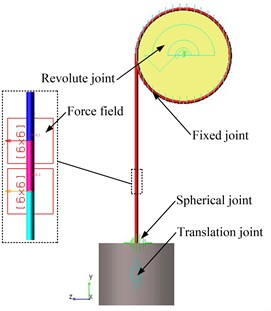## 4. Results and discussion

Consider a hoisting cable with $\rho =$ 0.4 kg/m, ${Q}_{1}=\mathrm{}$3.46×107 N, ${Q}_{23}=\mathrm{}$1.2×105 N∙m and ${Q}_{4}=\mathrm{}$660 N∙m2. The Moment of inertia $J$ and Hoisting mass ${m}_{c}$ are 2×10-5 kg∙m and 2000 kg. The total length $L$ of the hoisting cable is 244 m and the initial length $l\left(0\right)$ is 4 m. The vertical excitation applied to the head sheave is $e\left(t\right)=0.003\mathrm{s}\mathrm{i}\mathrm{n}\left(2\pi t\right)$ m. The maximum velocity and acceleration are 6 m/s and 2 m/s2, respectively. In the ADAMS simulation, the hoisting cable is discretized into 610 cylinders. The total simulation time is 50 s and the time step size is 0.01 s. The element number is $n=$30.

In order to validate the finite element model using the simulation results obtained from the ADAMS model, the axial and the torsional displacements of the lower end of the cable in both simulations are analyzed. The comparisons are shown in Fig. 4.

Fig. 4a) Comparisons on the axial and b) torsional displacements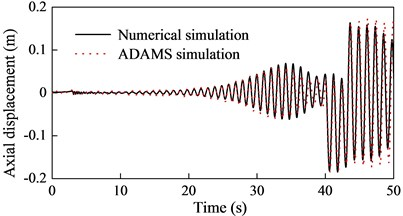a)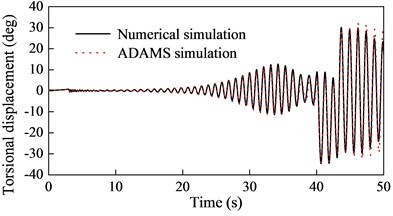b)

Fig. 4 shows that the numerical simulations are in reasonably good agreement with the ADAMS simulations, which confirms the validity of the finite element model. The curves demonstrate the longitudinal and torsional resonances in the system occur simultaneously.

In the following, the excitation frequency will be increased to 2 Hz, and then the longitudinal vibration of the lower end of the cable will be investigated with ${Q}_{23}=\mathrm{}$0 in case 1, ${Q}_{23}=\mathrm{}$0.9×105 in case 2 and ${Q}_{23}=\mathrm{}$1.2×105 in case 3. The results are shown in Fig. 5.

Fig. 5Longitudinal vibration with different Q23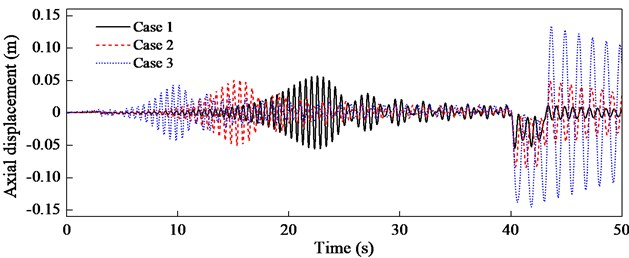Fig. 5 indicates both the resonance frequency and the maximum amplitude with different ${Q}_{23}$ are entirely different, which proves that the coupling effect of the cable itself has a great influence on the longitudinal vibration. Therefore, the coupling effect should be considered in practice for the accurate response prediction and vibration control. The resonance of the cable in case 2 occurs earlier than that in case 1 and later than case 2, which reveals that the coupling effect reduces the longitudinal natural frequency of the cable. In case 1 the maximum amplitude occurs at the deceleration stage while in case 3 it appears in the resonance region, which implies that the maximum amplitude will shift from the resonance region to the deceleration stage as the coupling coefficient increases. Besides, the major reason accounting for such large amplitude in case 3 is that the strand of cable will become looser or tighter when the cable is subjected to axial loading only, which mainly contributes to the additional length change.

## 5. Conclusions

The coupled axial-torsional responses of the hoisting cable with time-varying length are investigated in aspects of both theory and ADAMS simulation. The theoretical model of the coupled axial-torsional vibrations of the hoisting cable is established using the FEM with a variable-length element. An ADAMS simulation model is established in order to validate this theoretical model. The result shows that the numerical simulation is in reasonably good agreement with the ADAMS simulation. The analysis on the frequency of the cable indicates the coupling effect reduces the natural frequency of the cable and the maximum amplitude shifts from the resonance region to the deceleration stage as the coupling coefficient increases.

Accepted
25 September 2015
Published
09 October 2015
SUBJECTS
Mechanical vibrations and applications
Keywords
hoisting cable
coupled vibration
finite element method